# Diliberto-Straus algorithm

(diff) ← Older revision | Latest revision (diff) | Newer revision → (diff)

An algorithm first proposed in 1951 by S.P. Diliberto and E.G. Straus [a2]. It is concerned with the problem of finding a best approximation tofrom the subspace. In the original paper,andwere closed, bounded intervals in, but there is no difficulty in thinking of them as compact Hausdorff spaces, and then giving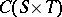the usual supremum norm (cf. also Approximation theory). The subspace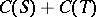might be more properly written as, where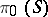is the subspace ofconsisting of functions that are constant on. For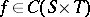, one definesandThe algorithm is then given by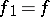,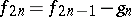and, where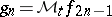and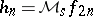.

Diliberto and Straus showed that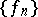is an equicontinuous family of functions (cf. also Equicontinuity) and that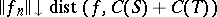. These two facts may be used to deduce, via the Ascoli theorem, that the sequencehas cluster points (cf. also Cluster set), and that any such cluster point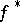has the property that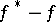is a closest point tofrom. These results are interesting, because they establish that everyhas a best approximation in the infinite-dimensional subspace. Diliberto and Straus could not establish that the sequenceis convergent. However, they came intriguingly near to such a proof when they apparently verified that in the algorithm the incremental functionsand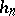satisfy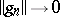and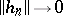as. Unfortunately, a referee demanded that the paper be shortened prior to publication, so the sequence of lemmas needed to establish this result appears without proof. Up till now (1998), no-one has been able to reproduce the series of arguments needed to support some of these lemmas. However, later work by G. Aumann [a1] established thatandasindependently of the methods of Diliberto and Straus. Armed with this knowledge, Aumann was able to prove that the Diliberto–Straus algorithm converges. A relatively simple proof of the convergence of the algorithm, which contains Aumann's result on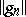and, can be found in [a6].

## Generalizations.

There are two natural generalizations of the Diliberto–Straus algorithm. First, one can continue working in the spacebut increase the complexity of the subspace fromto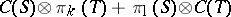. Here,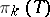is the subspace of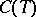consisting of all polynomials of degree at most, and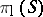has a similar definition. One has to notice now that the operator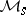as defined above has the property that, for each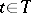, the numberis the best approximation to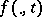from. In the present scenario, the correct generalization is to take the mapping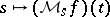to be the polynomial of degreewhich is the best approximation tofrom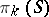. One can then examine the convergence of the algorithm as before. This was done by N. Dyn [a3], who showed that convergence cannot be guaranteed. In fact, if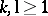, then there exists a functionsuch that the algorithm is stationary, that is,for all, but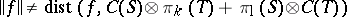.

Another very natural generalization is to consider the algorithm in different spaces. For example, one could examine the behaviour of the analogous algorithm in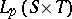, with the subspace, where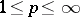. In the case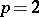, the algorithm takes place in a Hilbert space, and becomes the alternating algorithm of von Neumann. Because of the uniform convexity offor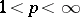, the analysis of the algorithm can be carried out in considerable generality. The case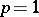is more delicate. As with the case studied by Dyn, there exist functions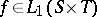for which the algorithm is stationary, but. However, a class of functions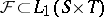can be identified on which the algorithm can be shown to converge. When working within this set of functions, the task of verifying that the incremental functions satisfy thatand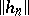tend tois actually easier than the original problem tackled by Aumann. These results can be found in [a4], [a5].

Finally, it was the intention of Diliberto and Straus to consider approximation of multivariate functions by sums of univariate functions, and their paper gives a number of such results. Other generalizations inare given in [a7].

How to Cite This Entry:
Diliberto-Straus algorithm. Encyclopedia of Mathematics. URL: http://encyclopediaofmath.org/index.php?title=Diliberto-Straus_algorithm&oldid=15787
This article was adapted from an original article by W. Light (originator), which appeared in Encyclopedia of Mathematics - ISBN 1402006098. See original article